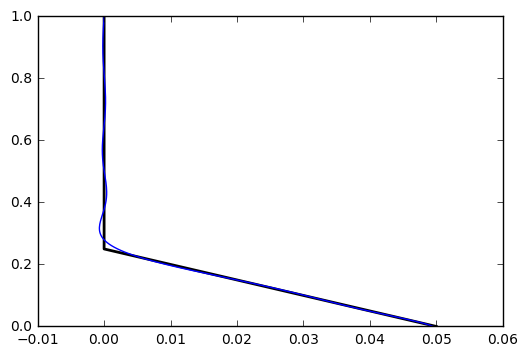# The Hanging Chain: Part 2¶

For mathematical details, see https://scipython.com/blog/the-hanging-chain-part-2/

Change the definition of the function f(u) to change the initial conditions of the chain.

In :
import numpy as np
from scipy.special import j0, j1, jn_zeros
import matplotlib.pyplot as plt
from matplotlib import animation
from IPython.display import HTML

In :
# Acceleration due to gravity, m.s-2
g = 9.81
# Chain length, m
L = 1
# Vertical axis (m)
N = 201
z = np.linspace(0, L, N)
# Scaled vertical axis
u = 2 * np.sqrt(z/g)

In :
# The initial state of the chain, x(z,0): linear from (0,d) to (c,0) then zero
# from (c,0) to (L,0).
d, c = 0.05, L/4
p, q = -d/c, d
def f(u):
z = u**2 * g / 4
if np.isscalar(z):
if z > c:
return 0
return p*z + q
h = p*z + q
h[z>c] = 0
return h

nmax = 10
# jn_zeros calculates the first n zeros of the zero-th order Bessel function of the first kind
w = jn_zeros(0, nmax)
b = 2 * np.sqrt(L/g)
def func(u, n):
return u * f(u) * j0(w[n-1] / b * u)
A = []
for n in range(1, nmax+1):
An = quad(func, 0, b, args=(n,)) * 2 / (b * j1(w[n-1]))**2
A.append(An)

In :
# To plot the initial condition of the chain, run this cell.
plt.plot(f(u), z, 'k', lw=2)
xfit = np.zeros(N)
for n in range(nmax):
xfit += A[n-1] * j0(w[n-1] / b * u)
plt.plot(xfit,z)
plt.show()In :
# Set up the Figure and Axes
fig, ax = plt.subplots(figsize=(4,6))

ax.axis('off')
ax.set_xlim((-2*d, 2*d))
ax.set_ylim((0, L))

line, = ax.plot([], [], 'k', dashes=[5,2], lw=3)

In :
# Initialization function for the animation
def init():
line.set_data([], [])
return (line,)

In :
# Delay between frames (ms)
interval = 1000/24
# Total length of animation (s)
anim_duration = 5
# Total number of frames in the animation
nframes = int(anim_duration * 1000 / interval)

# The animation function, to be called sequentially
def animate(i):
t = i * interval / 1000
x = np.zeros(N)
for n in range(0, nmax):
x += A[n-1] * j0(w[n-1] / b * u) * np.cos(w[n-1] * t)
line.set_data(x, z)
return (line,)

In :
# Set up the animation
anim = animation.FuncAnimation(fig, animate, init_func=init,
frames=nframes, interval=interval, blit=True)

In :
# To save the animation as a gif, run this cell.
anim.save('chain.gif', writer='imagemagick', fps=1000/interval)

In :
# To show the animation in a Jupyter Notebook cell, run this cell.
HTML(anim.to_html5_video())

Out: